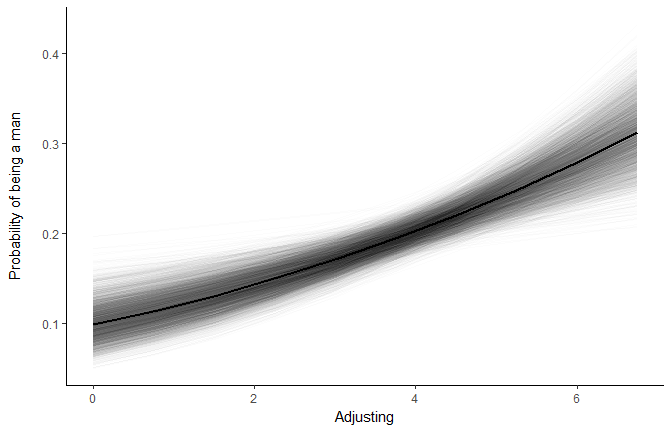03 Jun 2018 - Written by Dominique Makowski

# Fancy Plot (with Posterior Samples) for Bayesian Regressions

As Bayesian models usually generate a lot of samples (iterations), one could want to plot them as well, instead (or along) the posterior “summary” (with indices like the 90% HDI). This can be done quite easily by extracting all the iterations in `get_predicted` from the `psycho` package.

# The Model

``````# devtools::install_github("neuropsychology/psycho.R")  # Install the last psycho version if needed

library(tidyverse)
library(psycho)

# Import data
df <- psycho::affective

# Fit a logistic regression model
fit <- rstanarm::stan_glm(Sex ~ Adjusting, data=df, family = "binomial")
``````

We fitted a Bayesian logistic regression to predict the sex (W / M) with one’s ability to flexibly adjust to his/her emotional reaction.

# Plot

To visualize the model, the most neat way is to extract a “reference grid” (i.e., a theorethical dataframe with balanced data). Our refgrid is made of equally spaced predictor values. With it, we can make predictions using the previously fitted model. This will compute the median of the posterior prediction, as well as the 90% credible interval. However, we’re interested in keeping all the prediction samples (iterations). Note that `get_predicted` automatically transformed log odds ratios (the values in which the model is expressed) to probabilities, easier to apprehend.

``````# Generate a new refgrid
refgrid <- df %>%
psycho::refdata(length.out=10)

# Get predictions and keep iterations
predicted <- psycho::get_predicted(fit, newdata=refgrid, keep_iterations=TRUE)

# Reshape this dataframe to have iterations as factor
predicted <- predicted %>%
tidyr::gather(Iteration, Iteration_Value, starts_with("iter"))

# Plot all iterations with the median prediction
geom_line(aes(y=Iteration_Value, group=Iteration), size=0.3, alpha=0.01) +
geom_line(aes(y=Sex_Median), size=1) +
ylab("Probability of being a man\n") +
theme_classic()
``````# Credits

This package helped you? Don’t forget to cite the various packages you used :)

You can cite `psycho` as follows:

• Makowski, (2018). The psycho Package: an Efficient and Publishing-Oriented Workflow for Psychological Science. Journal of Open Source Software, 3(22), 470. https://doi.org/10.21105/joss.00470